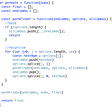# 561. Array Partition I

Given an integer array `nums` of `2n` integers, group these integers into `n` pairs `(a1, b1), (a2, b2), ..., (an, bn)` such that the sum of `min(ai, bi)` for all `i` is maximized. Return the maximized sum.

Example 1:

`Input: nums = [1,4,3,2]Output: 4Explanation: All possible pairings (ignoring the ordering of elements) are:1. (1, 4), (2, 3) -> min(1, 4) + min(2, 3) = 1 + 2 = 32. (1, 3), (2, 4) -> min(1, 3) + min(2, 4) = 1 + 2 = 33. (1, 2), (3, 4) -> min(1, 2) + min(3, 4) = 1 + 3 = 4So the maximum possible sum is 4.`

Example 2:

`Input: nums = [6,2,6,5,1,2]Output: 9Explanation: The optimal pairing is (2, 1), (2, 5), (6, 6). min(2, 1) + min(2, 5) + min(6, 6) = 1 + 2 + 6 = 9.`

Constraints:

• `1 <= n <= 104`
• `nums.length == 2 * n`
• `-104 <= nums[i] <= 104`

--

--

--

## More from Joo Hee Paige Kim

Consistency achieves everything https://github.com/paigekim29

Love podcasts or audiobooks? Learn on the go with our new app.

## How to Extract Entities using NLP in Swift## Different ways to start windows 10 into safe mode## APP scaling with operational excellence## Share Arduino code without actual code## Java Object Oriented Design — Java interface## Joo Hee Paige Kim

Consistency achieves everything https://github.com/paigekim29

## More from Medium## Algorithms: Recursion/BackTracking, Permutations## 3–Longest Substring Without Repeating Characters